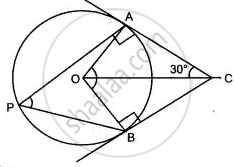Share

# In the Given Figure, O is the Centre of the Circle. Tangents at a and B Meet at C. If ∠Aco = 30°, Find: ∠Bco - Mathematics

Course

#### Question

In the given figure, O is the centre of the circle. Tangents at A and B meet at C. If ∠ACO = 30°, find: ∠BCO#### Solution

In the given fig, O is the centre of the circle and CA and CB are the tangents to the circle from
C. Also, ∠ACO = 30°
P is any point on the circle. P and PB are joined.
To find:  ∠ BCO
Proof:
In ΔOAC and OBC
OC = OC (Common)
OA = OB (radius of the circle)
CA = CB (tangents to the circle)
∴  ΔOAC ≅  ΔOBC (SSS congruence criterion)
∴ ∠ACO =  ∠BCO = 30°

Is there an error in this question or solution?